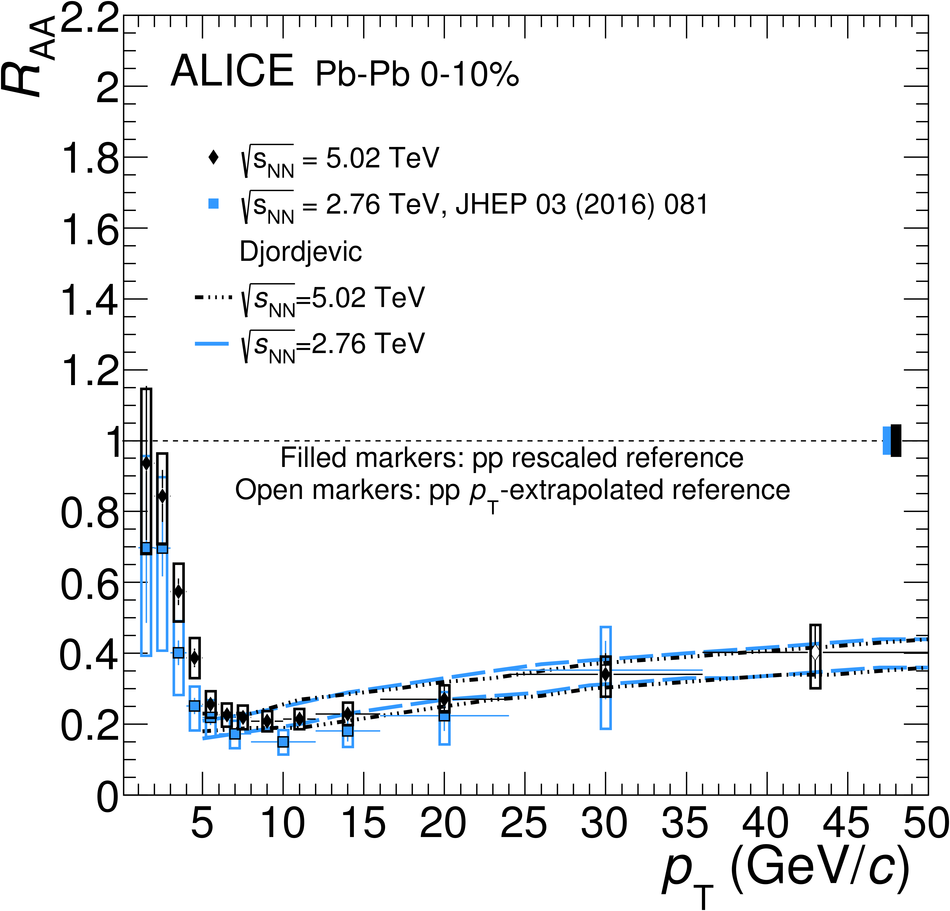# Figure 6

 Left panel: average $\RAA$ of prompt $\Dzero$, $\Dplus$ and $\Dstar$ mesons by ALICE compared to $\RAA$ of prompt $\Dzero$ mesons by CMS~ in the 0--10\% centrality class and at $\sqrtsNN= 5.02~\tev$. Statistical (bars), systematic (empty boxes), and normalisation (shaded box around unity) uncertainties are shown. Right panel: average $\RAA$ of $\Dzero$, $\Dplus$ and $\Dstar$ mesons compared with the Djordjevic model~ in the 0-10\% centrality class at two collision energies. Statistical (bars), systematic (empty boxes), and normalisation (shaded box) uncertainties are shown.